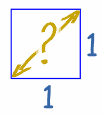# Irrational Numbers

An Irrational Number is a real number that cannot be written as a simple fraction.

Irrational means not RationalLet's look at what makes a number rational or irrational ...

### Rational Numbers

A Rational Number can be written as a Ratio of two integers (ie a simple fraction).

Example: 1.5 is rational, because it can be written as the ratio 3/2

Example: 7 is rational, because it can be written as the ratio 7/1

Example 0.333... (3 repeating) is also rational, because it can be written as the ratio 1/3

### Irrational Numbers

But some numbers cannot be written as a ratio of two integers ...

...they are called Irrational Numbers.

### Example: π (Pi) is a famous irrational number.π = 3.1415926535897932384626433832795... (and more)

We cannot write down a simple fraction that equals Pi.

The popular approximation of 22/7 = 3.1428571428571... is close but not accurate.

Another clue is that the decimal goes on forever without repeating.

## Cannot Be Written as a Fraction

It is irrational because it cannot be written as a ratio (or fraction),
not because it is crazy!

So we can tell if it is Rational or Irrational by trying to write the number as a simple fraction.

### Example: 9.5 can be written as a simple fraction like this:

9.5 = 192

So it is a rational number (and so is not irrational)

Here are some more examples:

Number   As a Fraction   Rational or
Irrational?
1.75   74   Rational
.001   11000   Rational
√2
(square root of 2)
?   Irrational !

## Square Root of 2

Let's look at the square root of 2 more closely.When we draw a square of size "1", what is the distance across the diagonal?

The answer is the square root of 2, which is 1.4142135623730950...(etc)

But it is not a number like 3, or five-thirds, or anything like that ...

... in fact we cannot write the square root of 2 using a ratio of two numbers

... I explain why on the Is It Irrational? page,

... and so we know it is an irrational number

## Famous Irrational NumbersPi is a famous irrational number. People have calculated Pi to over a quadrillion decimal places and still there is no pattern. The first few digits look like this:

3.1415926535897932384626433832795 (and more ...)The number e (Euler's Number) is another famous irrational number. People have also calculated e to lots of decimal places without any pattern showing. The first few digits look like this:

2.7182818284590452353602874713527 (and more ...)The Golden Ratio is an irrational number. The first few digits look like this:

1.61803398874989484820... (and more ...)Many square roots, cube roots, etc are also irrational numbers. Examples:

 √3 1.7320508075688772935274463415059 (etc) √99 9.9498743710661995473447982100121 (etc)

But √4 = 2 (rational), and √9 = 3 (rational) ...

... so not all roots are irrational.

### Note on Multiplying Irrational Numbers

Have a look at this:

• π × π = π2 is irrational
• But √2 × √2 = 2 is rational

So be careful ... multiplying irrational numbers might result in a rational number!

### Fun Facts ....

Apparently Hippasus (one of Pythagoras' students) discovered irrational numbers when trying to write the square root of 2 as a fraction (using geometry, it is thought). Instead he proved the square root of 2 could not be written as a fraction, so it is irrational.

But followers of Pythagoras could not accept the existence of irrational numbers, and it is said that Hippasus was drowned at sea as a punishment from the gods!